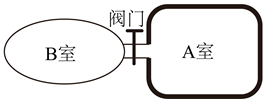（1）鱼通过增加 $\mathrm{B}$ 室体积获得大小为 $a$ 的加速度、需从 $\mathrm{A}$ 室充入 $\mathrm{B}$ 室的气体质量 $\Delta m$;
（2）鱼静止于水面下 $H_1$ 处时, B 室内气体质量 $m_1$ 。